# Getting the Moment X Rotation - Steel Bolted Connection

Hello,

I would like to plot a moment versus rotation diagram of a bolted connection, to compare with Eurocode-Part 3. Are there any people in the community that did some similar work in Ansys?

I have found a lot of articles and thesis, but neither of them say how they plot this graph. And example of article is attached.

I am using solid elements.

Thank you,

Regards.

Fabricio

«13

• You have to put the article in a zip file to attach it.

• edited May 2018

Hello Peter, I am sending the zip file, thank you very much!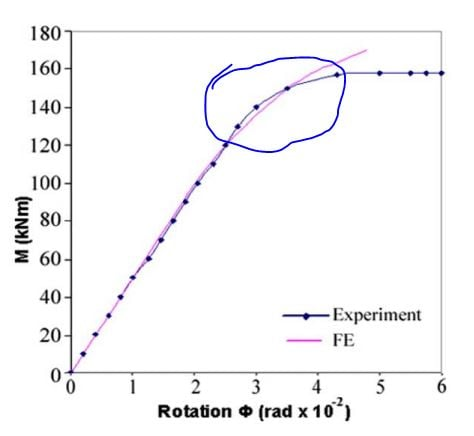The figure 10 is the diagram that I would like, I have difficult to find the point featured in blue. For you understand, now I am using the contact problems that you helped me, somedays ago. I am applying the geometric nonlinearity theory in Steel boled connectins.

Regards,

Fabricio

• It seems rotation is really vertical deformation divided by cantilever length.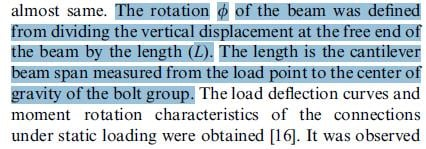That seems simple enough.

• edited May 2018

Thank you for your promt response Peter.

Yes It is simple, but is there anway using like "User defined Result", to write the equation: M (F * Lbeam) in Y axis, and r (Uz / Lbeam) in X axis?

I am looking for it, despite this I am using Excel. But I think that it is possible. I have read some article that was written it, but I could not find the article or site web where I have read.

Thank you, very much.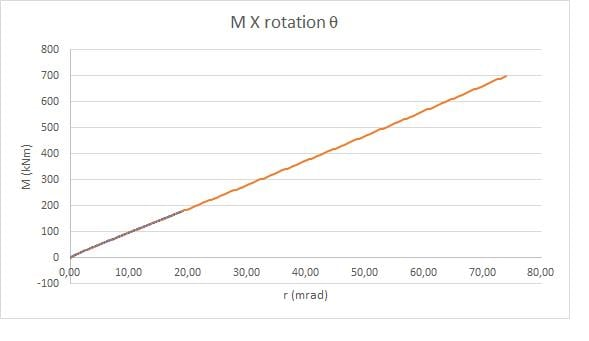The question is about  the point featured in blue in the post before. Because my diagram seems to be linear, when it had to be nonlinear, because the contact. The beam that I am using, is W200X80, the beam Mp = 406,9kNm. I would like to do this nonlinearity, when is near of tensile strength.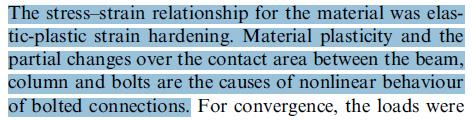• edited May 2018

No I have not. Do you you think that is mandatory/indispensable?

• edited May 2018

I think that, that problem may be it. But my teacher comments that the geometric nonlinearity has to appear without the material plasticity, I became in doubt with this comments.

• Plasticity will dominate the nonlinearity. You can run the same model with and without plasticity and show your teacher that plasticity causes a very large change in the shape of the curve.

• edited May 2018

Good Peter! I will do it to show her.

Another doubt about Moment X Rotation in Ansys. For the picture below: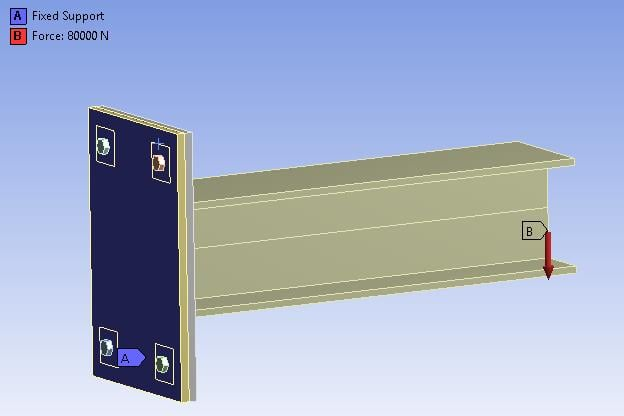The beam length is 1 meter. So the moment will be the force applied. But if I calculate the plate stress:

S = -M/W = -6*M/B*L²

The plate is 400mm X 600 mm. So the stress will be -6*M/(144000cm&sup2. For example, force of 24 kN, the minimum plate stress wil be 1 MPa. But the Ansys results were 66,154MPa. So there is something wrong.

I think that the force applied in Area, maybe give differents results. The stress display option was average.

What could be wrong?

Thank you very much again Peter!

• Fabricio,

I don't recognize the equations you used above.

The geometry of this model does not fit any simple equation you can find in a textbook.

Trying to use simple equations on geometry that is not simple is what is wrong.

Regards,
Peter

• edited May 2018

Peter, I am trying to calibrate the model, that this why I am comparing with some simple equations. For example a cantilever beam displacement by the beam theory. A cantilever beam with a concentrade load in the extreme, has the max displacement: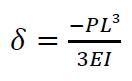So E= 20000 kN/cm² I = 12550 cm^4 (approximated, beacuse I am using a commercial steel)

With the model I am finding 17mm the maximum displacement in Z, but with the equation is 2mm. So ten times more. I am looking for the error in the model, but I am not finding. Can you help me?I am not working with simmetry, beacuse in the future, the load will not bee simmetric.

I plotted the graph below to compare, maybe it is easier o understand my difficulty.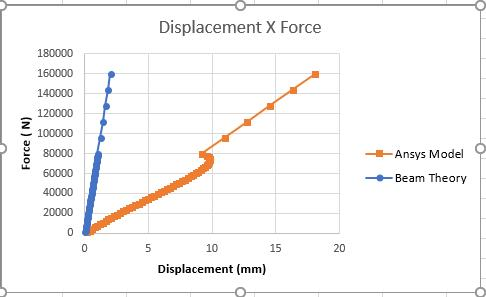The model iss attached. I did not attach the complet model, because It would be so big. So I did not attach the results.

Thank you very much!!

• edited May 2018

Sorry

L = 100cm. I forgot to write the beam length.

• Fabricio,

The ANSYS model includes a flexible baseplate and a flexible beam. They both contribute to the tip deflection.
The illustration below is not to scale, but you get the point that the majority of the tip displacement is due to baseplate flexibility.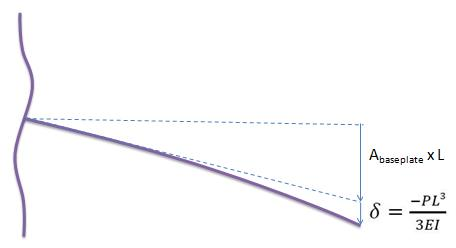If you use a fixed support on the edges at the base of the beam instead of the bolts, then the ANSYS model will give you a very similar displacement to the cantilever beam equation, which assumes a perfectly rigid baseplate.

• edited May 2018

Peter,

In the graph below, the "Bisection Occured" means the moment at which the plastification started, doesn't it?In other words, the yield strength was reached.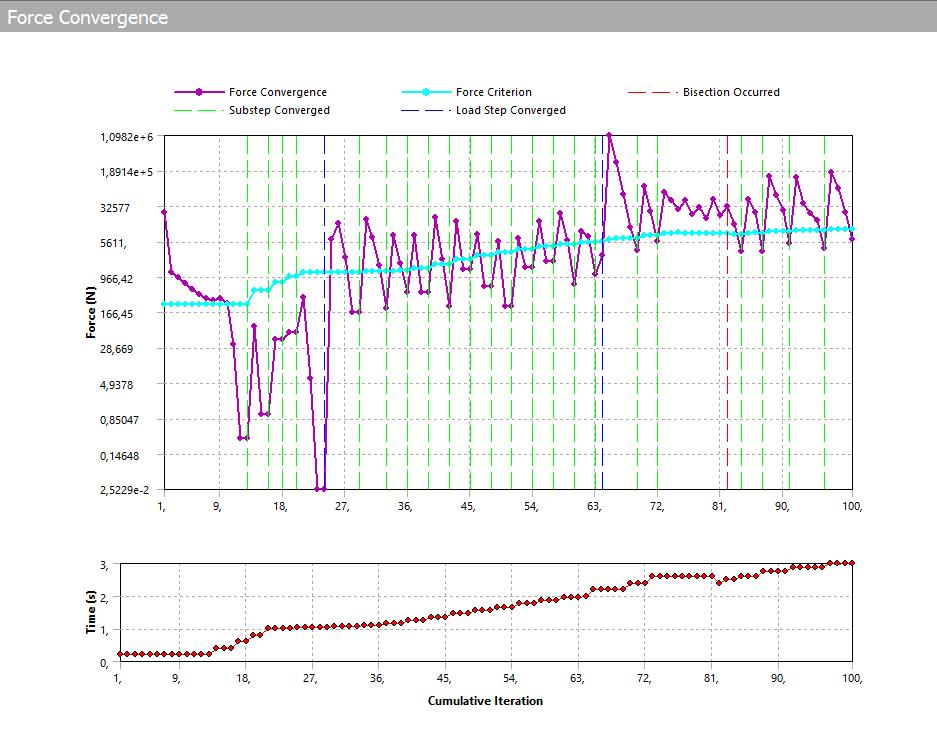Thank you!

• You can get bisection in a model without plasticity. It is not necessarily linked.

I will oversimplify this to describe it briefly. In a nonlinear analysis, a small portion of the total load, say 20% is first attempted, which is called a substep. The solver inverts the stiffness matrix to solve for the unknown displacements, then plugs them back in to see if the force is within a small tolerance of being in equilibrium. That is called an iteration. If the tolerance was exceeded, the solver updates the stiffness matrix and inverts it to solve for revised unknown displacements and repeats the check for being in equilibrium. In your image above, it took 12 iterations for the force error to drop below the tolerance, which is called the convergence criterion and we say the substep has converged. Having successfully solved the first 20% of the load, it attempts to solve the second 20% of the load (the next substep), and the whole iteration process repeats. The second substep took only 3 iterations.

The software has rules built-in to prevent it from iterating too many times, trying to find a set of displacements within the force equilibrium tolerance. For example, it might take 49 iterations to converge on a 20% load increment, but instead, after 10 iterations, the software changes to a 10% load increment and starts a new set of iterations. That is called a bisection. It cuts the load increment in half. Then it uses 3 iterations to find equilibrium for the first 10% load increment, and it increments another 10% and uses another 3 iterations to find equilibrium for that substep.  So in this example, it took 16 iterations to attempt 20%, give up, then succeed at 10% twice in 3 iterations each. This is better than taking 49 iterations to get 20%, but if you could have told it to take 10% in the first place, it would have only taken 6 iterations.

• edited May 2018

Peter, thank you. Now "bisection occured" make sense.

But I have difficult to understand, using bolt pre-tension, when the plastification starts. I lock the pre-tension load in the first step. Then I use the model load, and increase subtep by substep.

But if I analyse the bolt normal tension, in the begining of the second step, it reached the plastification, I think that it occured because of bolt pre-tension.

• In step 1, you ramp on the tension force in the bolt shank. If the pretension force is high enough, you can get plastic strain in the model at step 1. You can plot plastic strain and find out where that is happening and by how much. If you don't want plastic strain in step 1, then change the material, the pretension or the geometry.

In step 2, the bolt length is locked, while the load is applied to the structure. You can have a second plot for time=2 for plastic strain to see how much that changed after the structural load was applied.

• edited May 2018

Ok.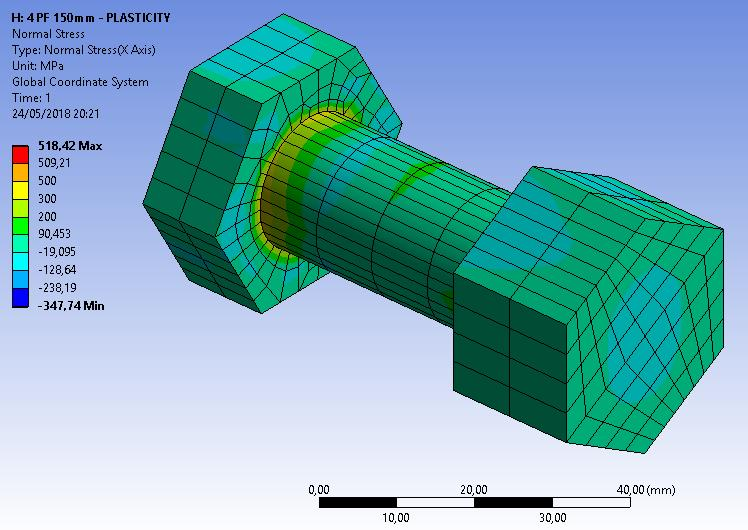But as you see in the picture, in the step 1, the bolt normal stress is high, almost the yield stress. So when the load is applied, the yield stress is reached in the first substeps. So with low load, the bolt plasticity is reached. You can see what I am trying to say in the picture below: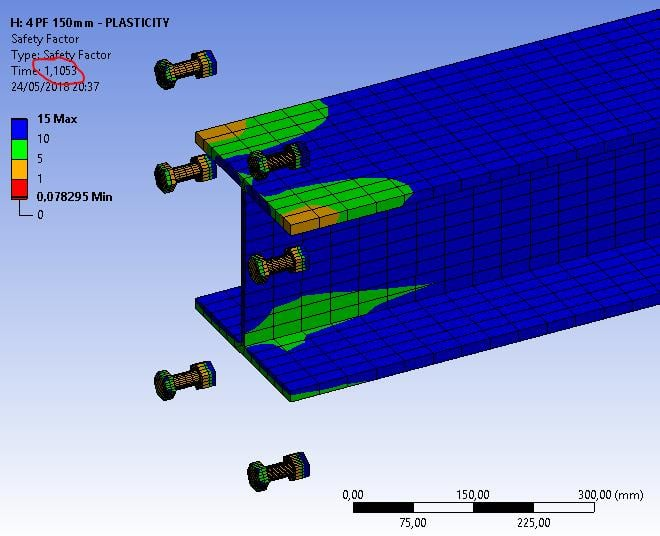• The bolts are pretensioned to nearly the yield point. That is good and correct.

Then the load is applied. The stress of the parts approaches yield. That is also fine.

It seems you are done. What is your question?

• edited May 2018

There is something wrong, because the stress of the parts approaches yield so early, with a very very low load.

The question is, sorry if it is obvious, but if the pretension is nearly the yield stress, the next load applied will do the bolt starts the plasticity, won't it?

Thank you!